• pytorch RNN:循环神经网络 代码详解 注释详细
2020-09-23 14:24:06
import torch
from torch import nn, optim
from torchvision import transforms
from torchvision import datasets

# 定义超参数
batch_size = 100
learning_rate = 1e-3
num_epoches = 2

# 下载训练集 MNIST 手写数字训练集
train_dataset = datasets.MNIST(

test_dataset = datasets.MNIST(
root='F:/PycharmProjects/pytorch-beginner-master/02-Logistic Regression/data', train=False, transform=transforms.ToTensor())

# 定义 Recurrent Network 模型
class Rnn(nn.Module):
def __init__(self, in_dim, hidden_dim, n_layer, n_class):
super(Rnn, self).__init__()
self.n_layer = n_layer
self.hidden_dim = hidden_dim
#输入[seq_len=100,batch_size=28,input_size=28] 输出为(seq_len=100,batch_size=28,num_directions*hidden_size) hidden_size = hidden_dim=128
self.lstm = nn.LSTM(in_dim, hidden_dim, n_layer, batch_first=True)
self.classifier = nn.Linear(hidden_dim, n_class)#输入为[100,128]输出为：[100,10]

def forward(self, x):
out, _ = self.lstm(x)#out=[100,28,128]
out = out[:, -1, :]#out[100,128]
out = self.classifier(out)#out=[100,10]
return out

model = Rnn(28, 128, 2, 10)  # 图片大小是28x28
use_gpu = torch.cuda.is_available()  # 判断是否有GPU加速
if use_gpu:
model = model.cuda()
# 定义loss和optimizer
criterion = nn.CrossEntropyLoss()

# 开始训练
for epoch in range(num_epoches):
print('epoch {}'.format(epoch + 1))
print('*' * 10)
model.train()
for i, data in enumerate(train_loader, 1):
img, label = data
#b, c, h, w = img.size()
#assert c == 1, 'channel must be 1'
img = img.squeeze(1)#将输入张量形状中的1 去除 比如输入为[2,1,3,5,1]的5维矩阵，去掉1后变为[2,3,5]的3维矩阵
# img = img.view(b*h, w)
# img = torch.transpose(img, 1, 0)
# img = img.contiguous().view(w, b, -1)
if use_gpu:
img = Variable(img).cuda()
label = Variable(label).cuda()
else:
img = Variable(img)
label = Variable(label)
# 向前传播
out = model(img)#输入[seq_len=100,batch_size=28,input_size=28]
loss = criterion(out, label)

# 向后传播
loss.backward()
optimizer.step()

# if i % 300 == 0:
#     print("loss: ",  loss.data.item())
print("loss: ", loss.data.item())

model.eval()
img, label = data
b, c, h, w = img.size()
assert c == 1, 'channel must be 1'
img = img.squeeze(1)
# img = img.view(b*h, w)
# img = torch.transpose(img, 1, 0)
# img = img.contiguous().view(w, b, h)
if use_gpu:
img = Variable(img).cuda()
label = Variable(label).cuda()
else:
img = Variable(img)
label = Variable(label)
out = model(img)
loss = criterion(out, label)
print("test loss: ",  loss.data.item())

更多相关内容
• 如果你看懂了我的上面的烂文。就明白EVst是核心。它们包含的数据到底有什么意义呢？E对每个单词有一个100维的矢量。用来保存每个单词的特征值。V对每个单词有一个100维的矢量。是计算输出单词概率的权值。...
• 简单理解RNN循环神经网络RNN(Recurrent Neural Network）介绍图说RNN宏观结构图说RNN微观结构 RNN(Recurrent Neural Network）介绍 介绍： RNN，也就是循环神经网络，是用来建模序列化数据的一种主流的深度学习模型...
• import tensorflow as tf from tensorflow.examples.tutorials.mnist import input_data mnist=input_data.read_data_sets('mnist_data/',one_hot=True) #注意这里用了one_hot表示，标签的形状是(batch_size,num_...
• 深度学习神经网络之循环神经网络RNN）Matlab实现循环神经网络RNN
• 循环神经网络代码RNN-超全注释 #inputs t时刻序列，也就是相当于输入 #targets t+1时刻序列，也就是相当于输出 #hprev t-1时刻的隐藏层神经元激活值 def lossFun(inputs, targets, hprev): xs, hs, ys, ps = {},...
•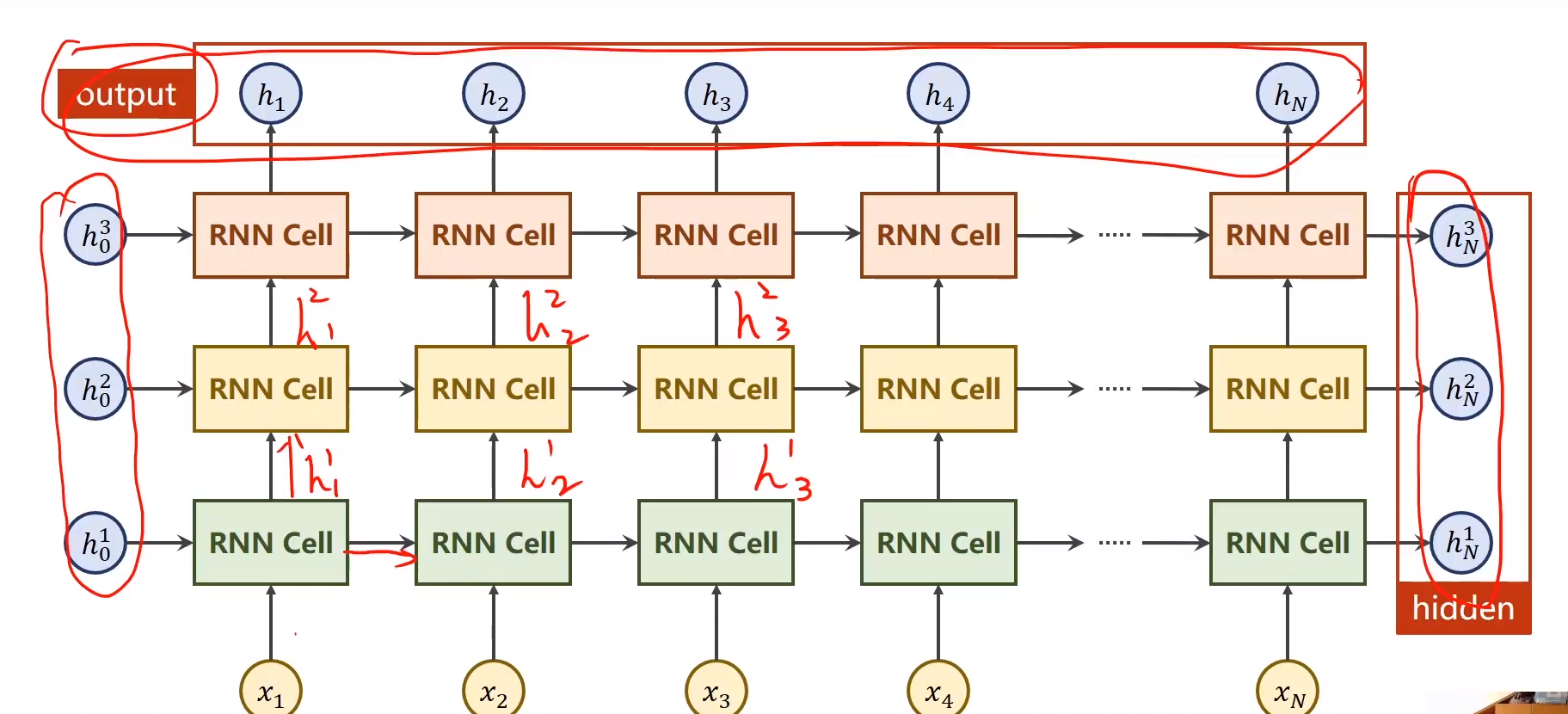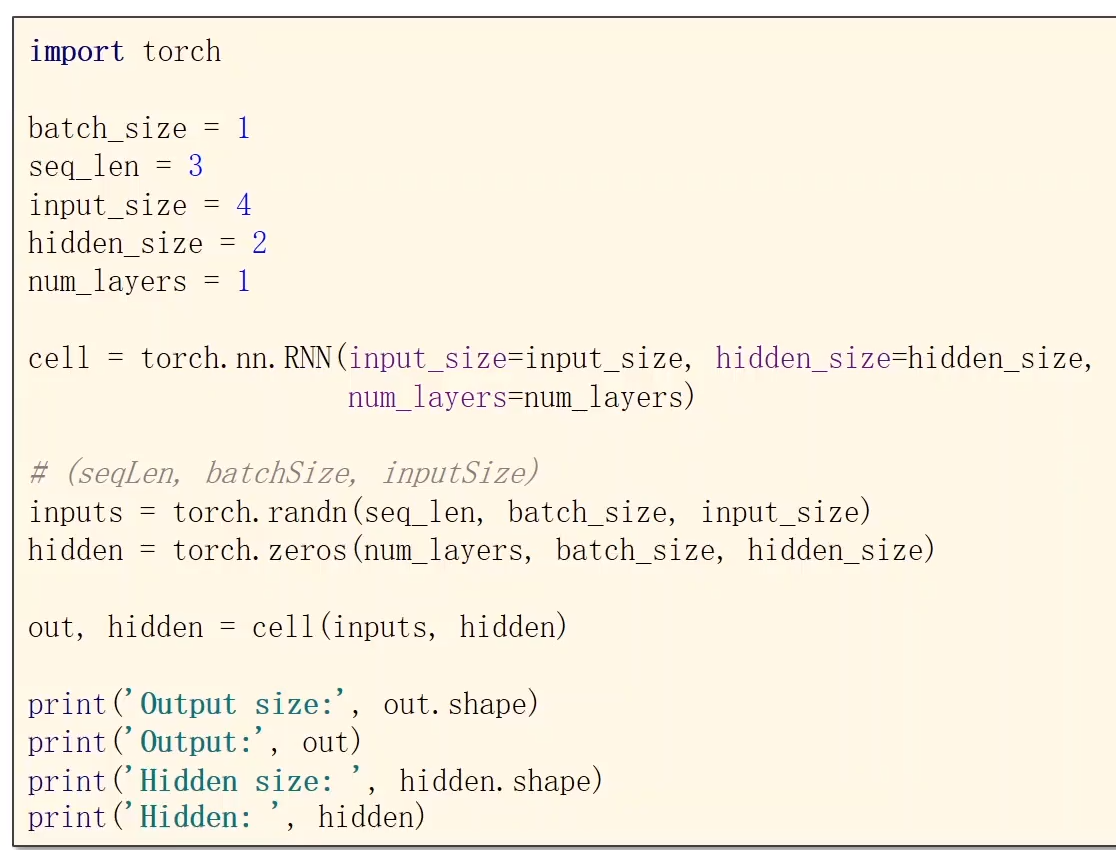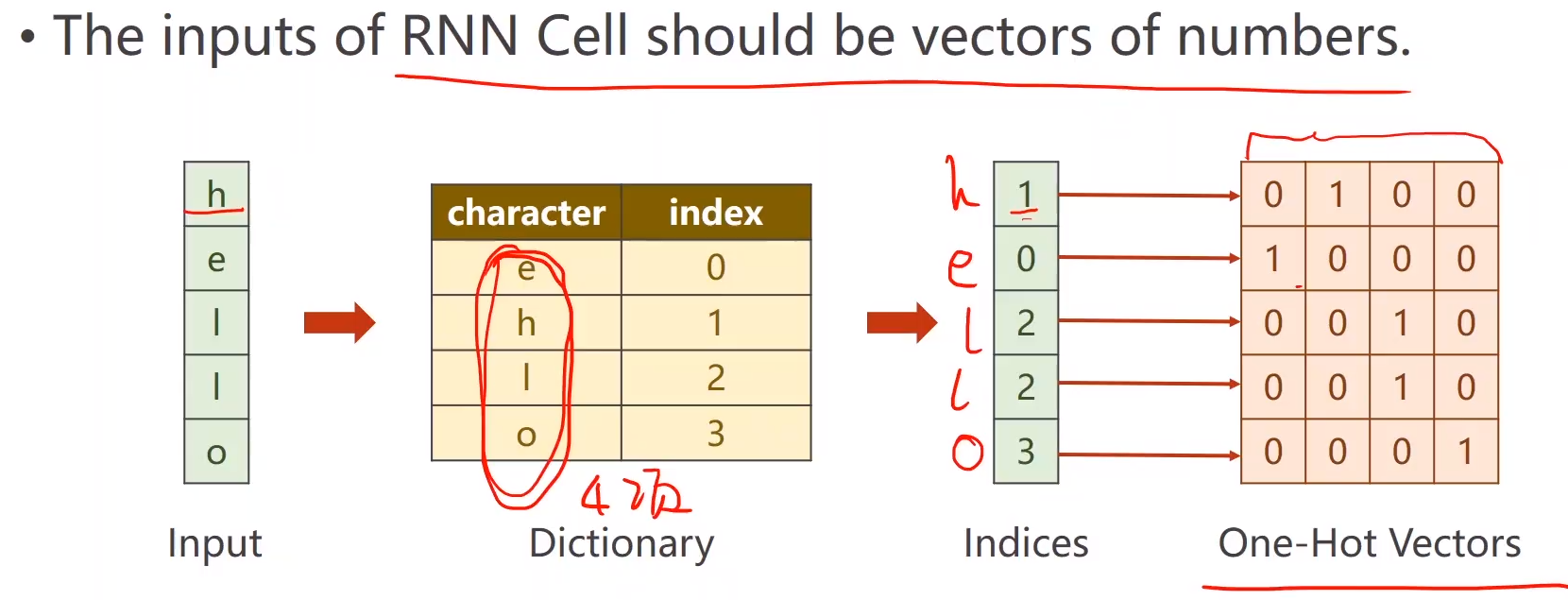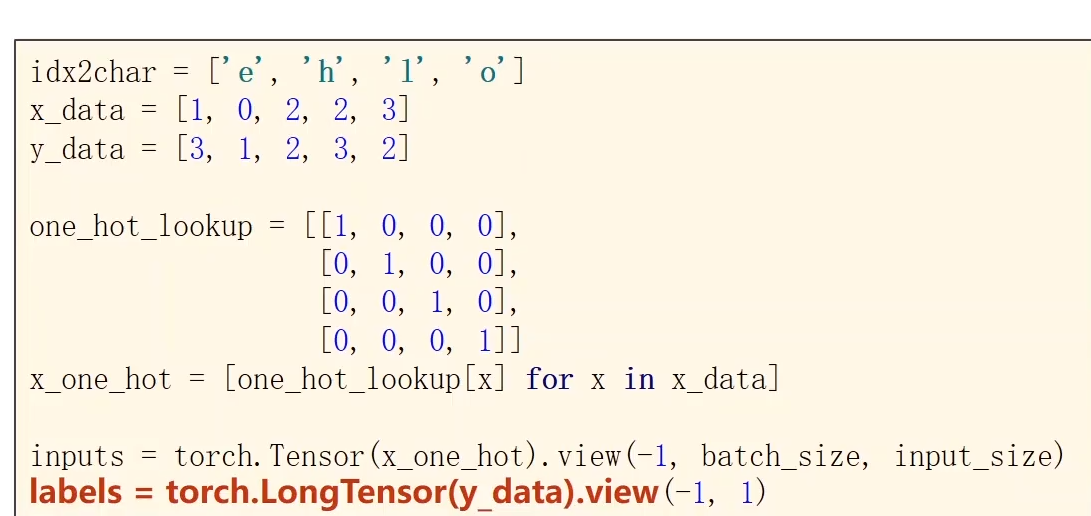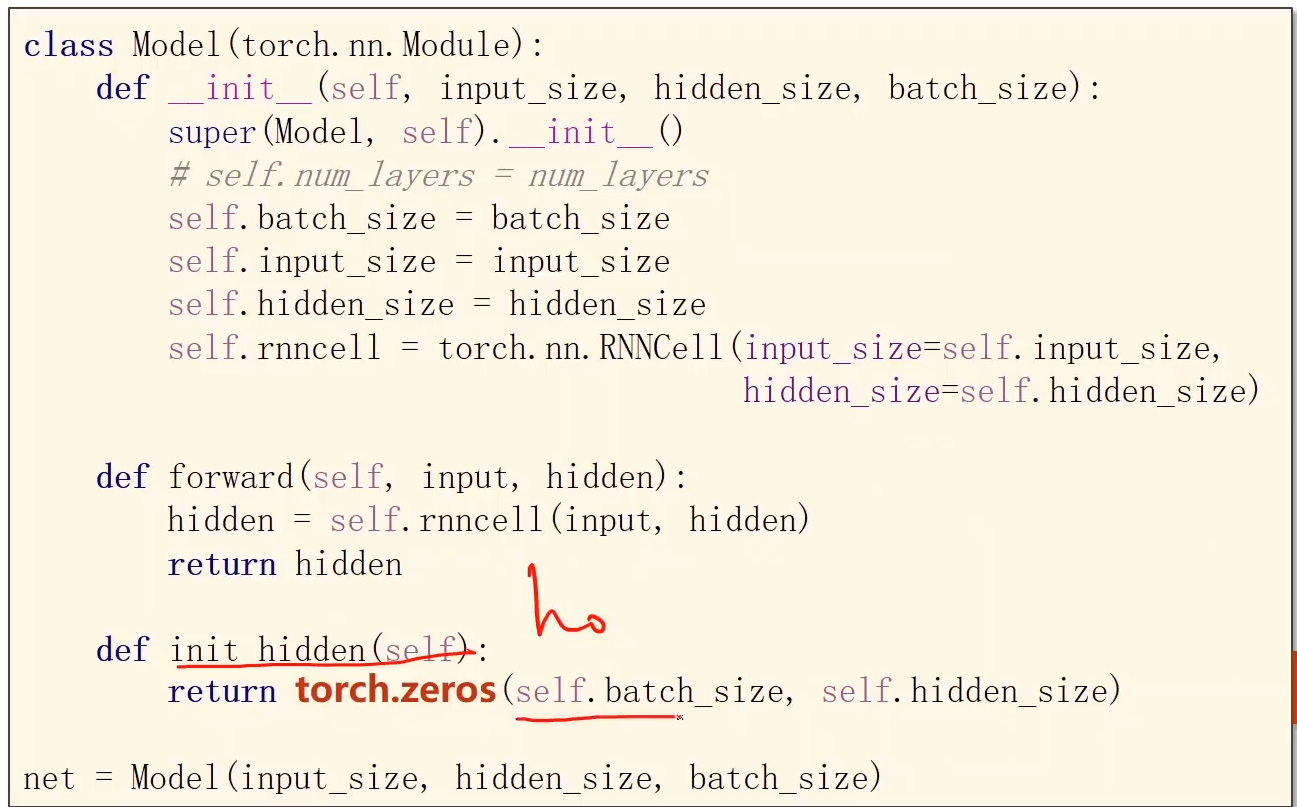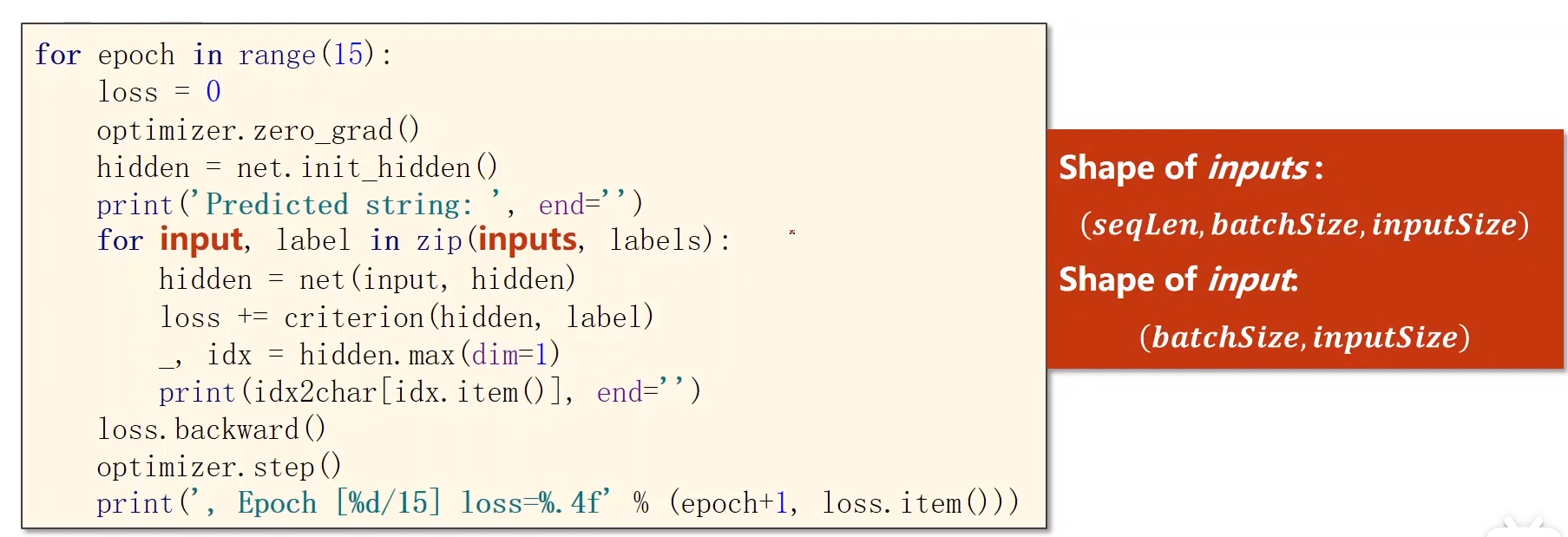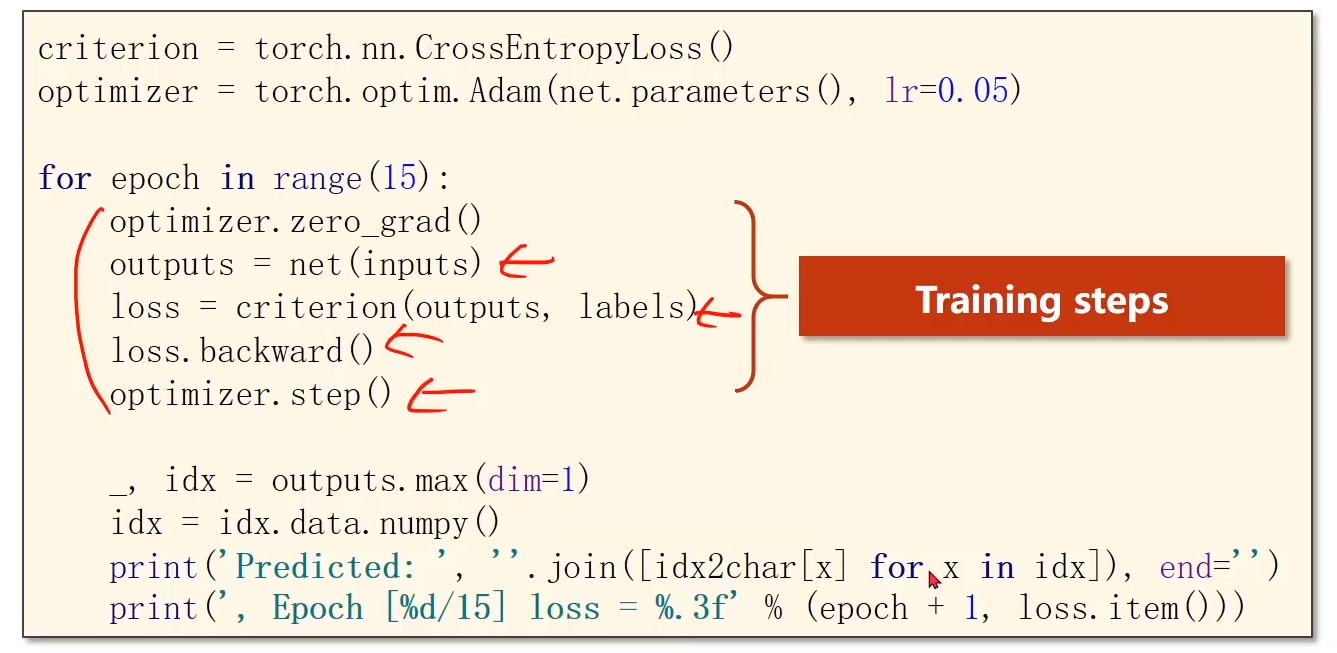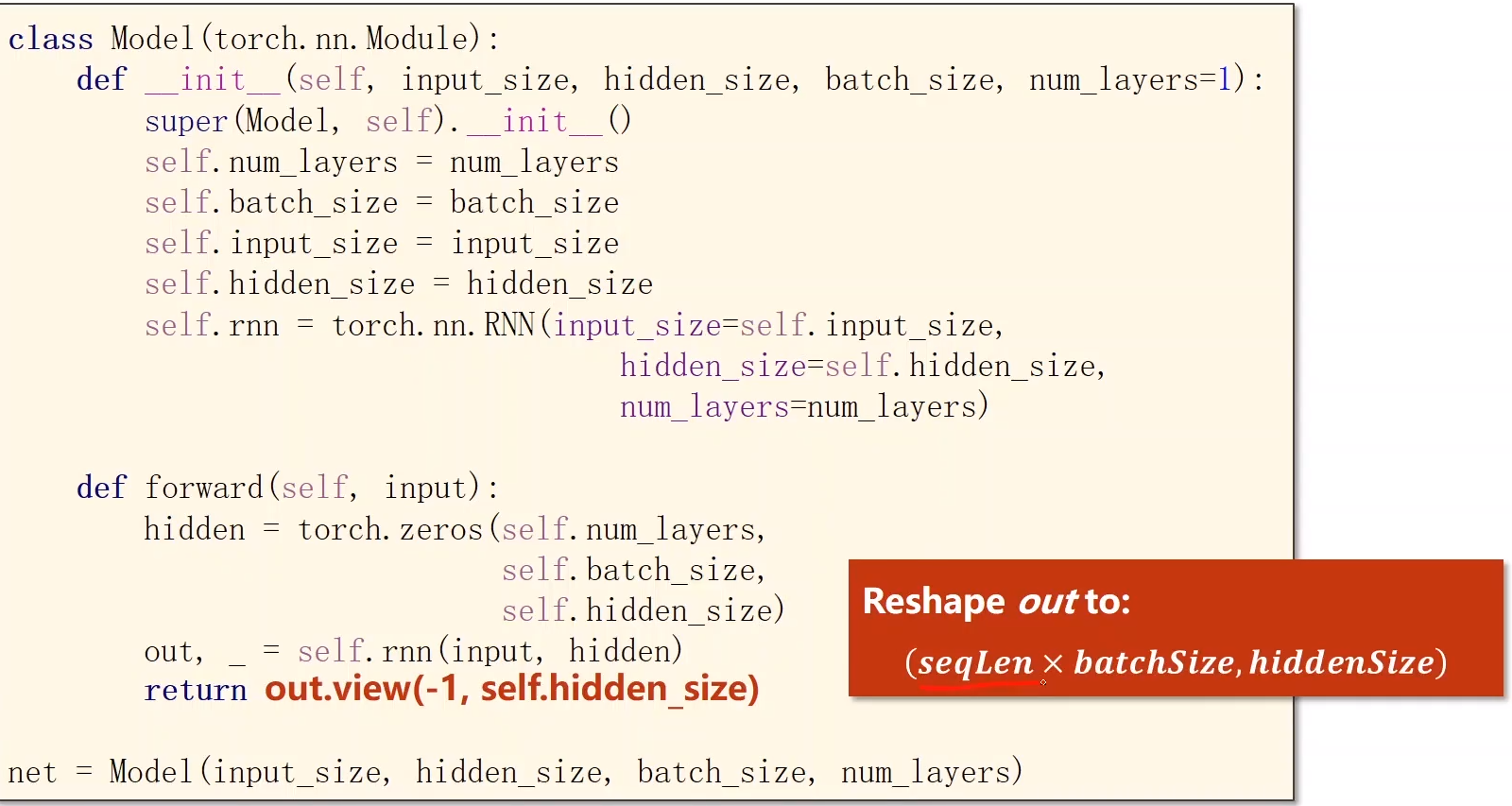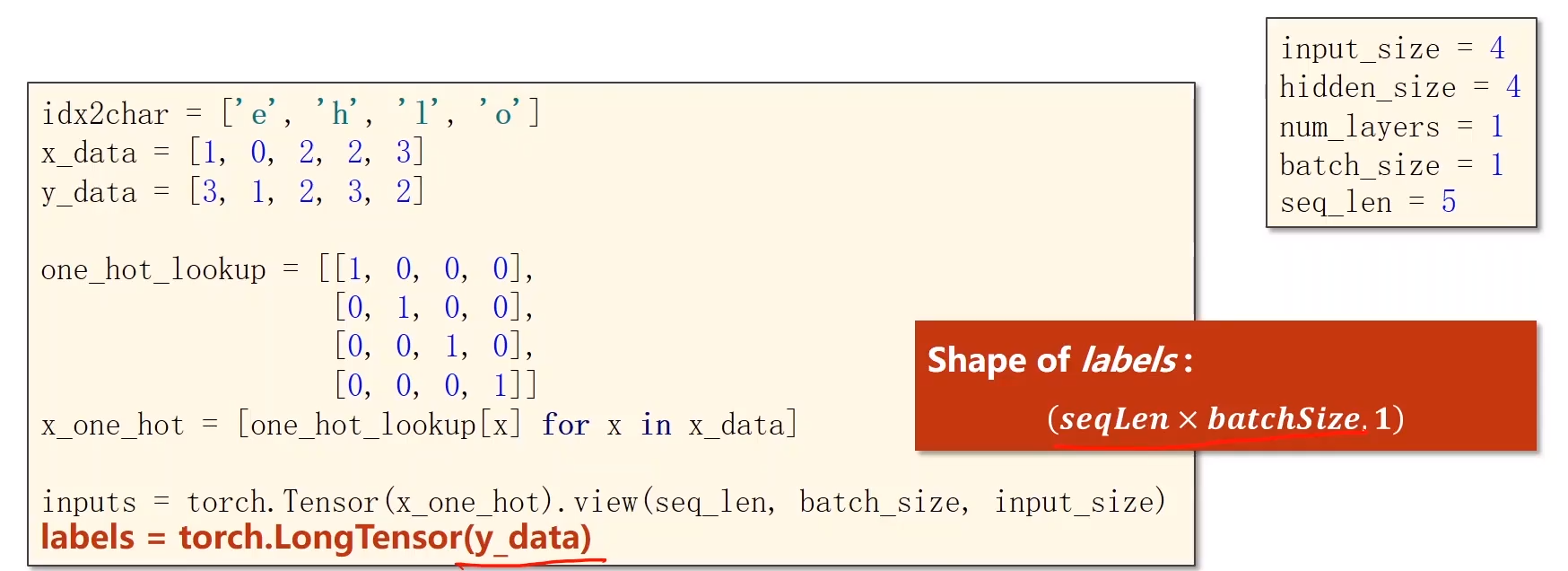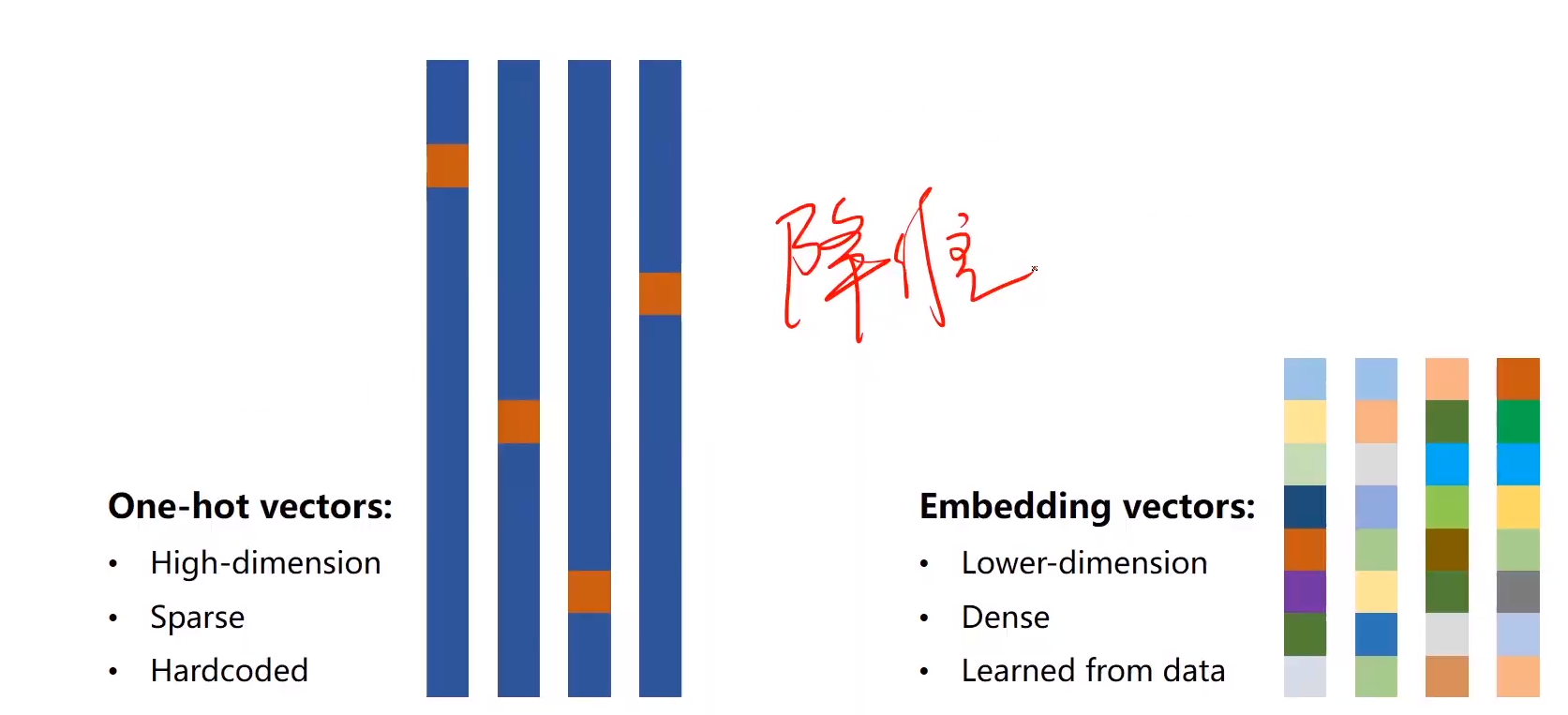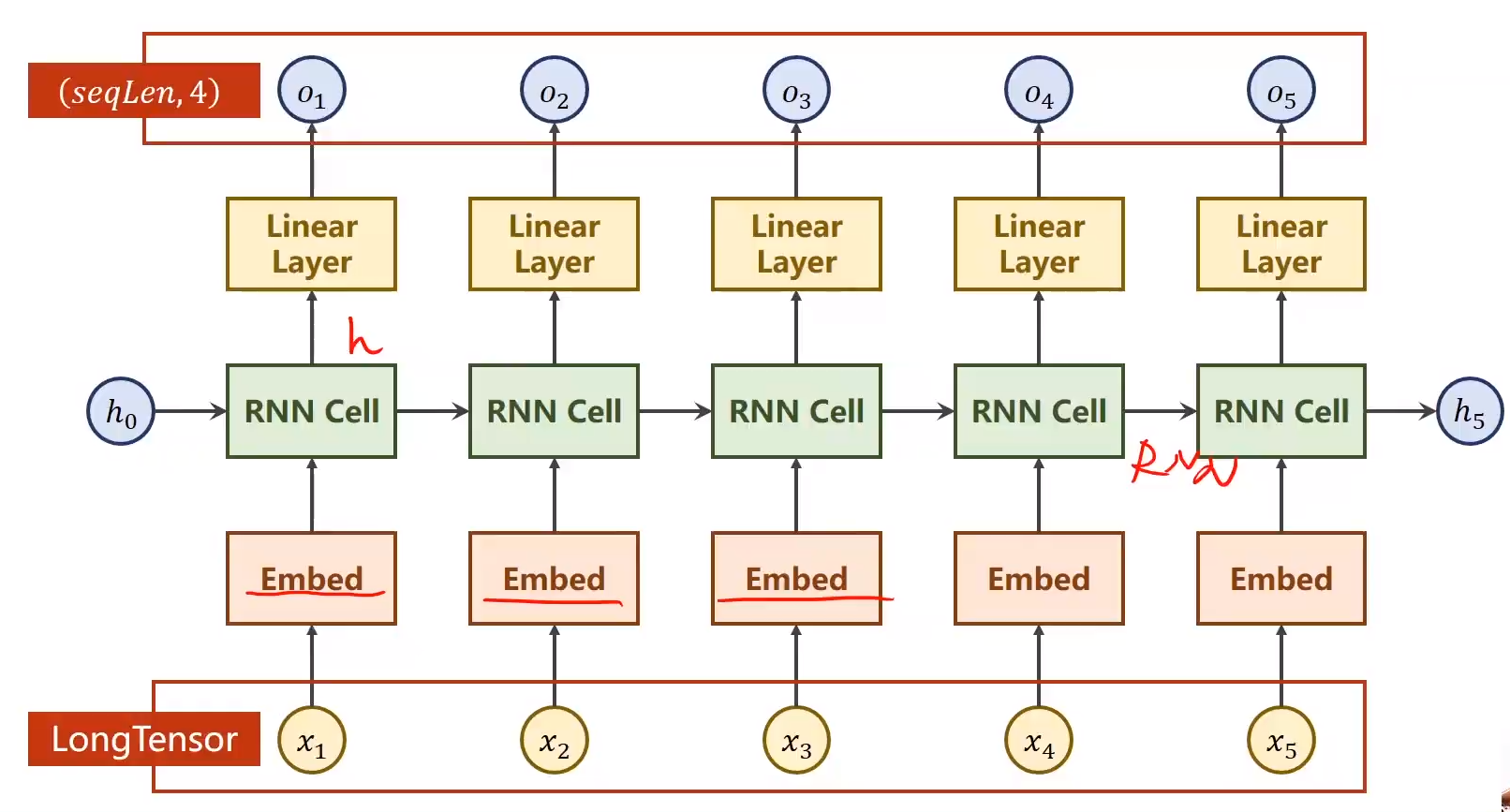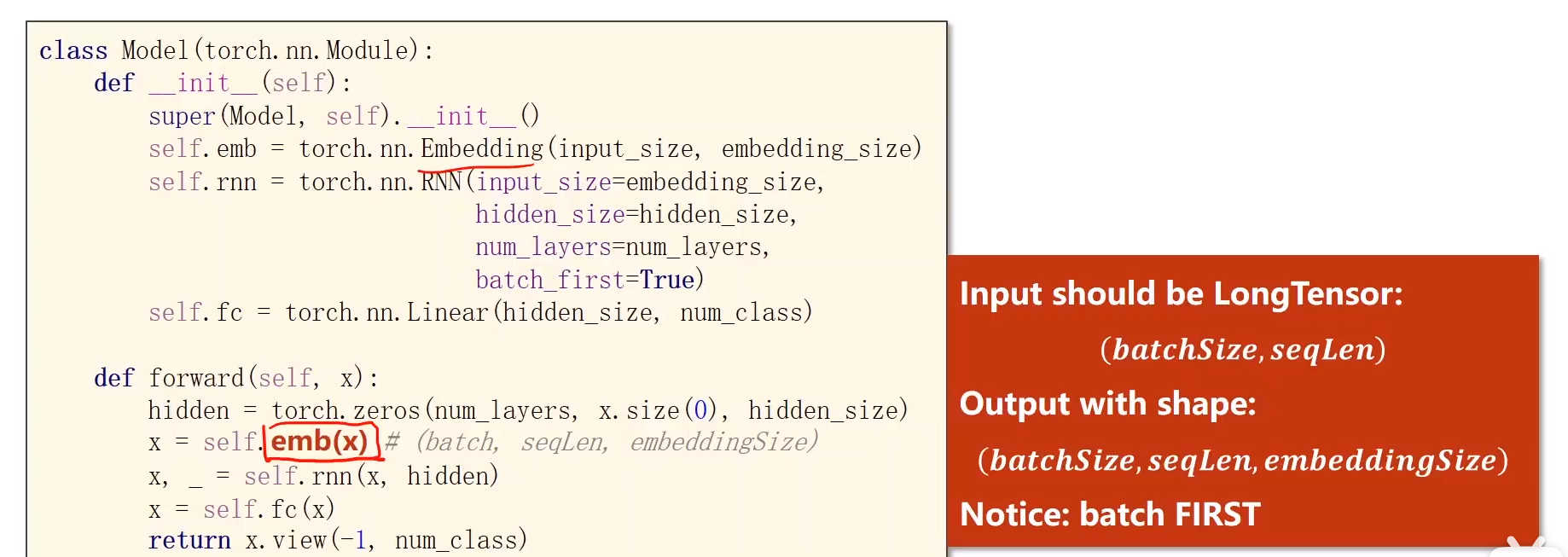展开全文• 循环神经网络RNN）的搭建，附带例子和完整代码

# 问题：

用RNN实现输入一个字母，预测出下一个字母（字母使用独热编码）：
输入a，预测出b
输入b，预测出c
输入c，预测出d
输入d，预测出e
输入e，预测出a

# 分析：

1.import
2.train,test:需要把输入的字母转换成独热编码，然后乱序，接着把x_train给reshape一下，转换成符合SimpleRNN的要求[送入样本数，循环核时间展开步数，每个时间步输入特征个数]
3.model=tf.keras.Sequential([…])
4.model.compile(…)
5.断点续训+模型保存+model.fit(…)
6.model.summary()
7.把训练的参数打印到txt文件中
8.acc/loss可视化
9.基于保存的模型进行预测

# 完整代码：

import numpy as np
import tensorflow as tf
from tensorflow.keras.layers import Dense, SimpleRNN
import matplotlib.pyplot as plt
import os

input_word = "abcde"
w_to_id = {'a': 0, 'b': 1, 'c': 2, 'd': 3, 'e': 4}  # 单词映射到数值id的词典
id_to_onehot = {0: [1., 0., 0., 0., 0.], 1: [0., 1., 0., 0., 0.], 2: [0., 0., 1., 0., 0.], 3: [0., 0., 0., 1., 0.],
4: [0., 0., 0., 0., 1.]}  # id编码为one-hot

x_train = [id_to_onehot[w_to_id['a']], id_to_onehot[w_to_id['b']], id_to_onehot[w_to_id['c']],
id_to_onehot[w_to_id['d']], id_to_onehot[w_to_id['e']]]
y_train = [w_to_id['b'], w_to_id['c'], w_to_id['d'], w_to_id['e'], w_to_id['a']]

np.random.seed(7)
np.random.shuffle(x_train)
np.random.seed(7)
np.random.shuffle(y_train)
tf.random.set_seed(7)

# 使x_train符合SimpleRNN输入要求：[送入样本数， 循环核时间展开步数， 每个时间步输入特征个数]。
# 此处整个数据集送入，送入样本数为len(x_train)；输入1个字母出结果，循环核时间展开步数为1; 表示为独热码有5个输入特征，每个时间步输入特征个数为5
x_train = np.reshape(x_train, (len(x_train), 1, 5))
y_train = np.array(y_train)

model = tf.keras.Sequential([
SimpleRNN(3),  # 记忆体个数越多，记忆力越好，但是同时占用资源会更多
Dense(5, activation='softmax')
])

loss=tf.keras.losses.SparseCategoricalCrossentropy(from_logits=False),
metrics=['sparse_categorical_accuracy'])

checkpoint_save_path = "./checkpoint/rnn_onehot_1pre1.ckpt"

if os.path.exists(checkpoint_save_path + '.index'):

cp_callback = tf.keras.callbacks.ModelCheckpoint(filepath=checkpoint_save_path,
save_weights_only=True,
save_best_only=True,
monitor='loss')  # 由于fit没有给出测试集，不计算测试集准确率，根据loss，保存最优模型

history = model.fit(x_train, y_train, batch_size=32, epochs=100, callbacks=[cp_callback])

model.summary()

# print(model.trainable_variables)
file = open('./weights.txt', 'w')  # 参数提取
for v in model.trainable_variables:
file.write(str(v.name) + '\n')
file.write(str(v.shape) + '\n')
file.write(str(v.numpy()) + '\n')
file.close()

###############################################    show   ###############################################

# 显示训练集和验证集的acc和loss曲线
acc = history.history['sparse_categorical_accuracy']
loss = history.history['loss']

plt.subplot(1, 2, 1)
plt.plot(acc, label='Training Accuracy')
plt.title('Training Accuracy')
plt.legend()

plt.subplot(1, 2, 2)
plt.plot(loss, label='Training Loss')
plt.title('Training Loss')
plt.legend()
plt.show()

############### predict #############

preNum = int(input("input the number of test alphabet:"))
for i in range(preNum):
alphabet1 = input("input test alphabet:")
alphabet = [id_to_onehot[w_to_id[alphabet1]]]
# 使alphabet符合SimpleRNN输入要求：[送入样本数， 循环核时间展开步数， 每个时间步输入特征个数]。此处验证效果送入了1个样本，送入样本数为1；输入1个字母出结果，所以循环核时间展开步数为1; 表示为独热码有5个输入特征，每个时间步输入特征个数为5
alphabet = np.reshape(alphabet, (1, 1, 5))
result = model.predict([alphabet])
pred = tf.argmax(result, axis=1)
pred = int(pred)
tf.print(alphabet1 + '->' + input_word[pred])



注：本文来自于中国大学mooc中北京大学的人工智能实践：Tensorflow笔记，在此感谢北大的曹健老师

展开全文python tensorflow keras 神经网络
• 一、循环神经网络 它并非刚性地记忆所有固定长度的序列，而是通过隐藏状态来存储之前时间步的信息。 我们想象现在有一组序列数据 data 0,1,2,3。 在当预测 result0 的时候,我们基于的是 data0, 同样在预测其他数据...

# 一、循环神经网络

它并非刚性地记忆所有固定长度的序列，而是通过隐藏状态来存储之前时间步的信息。

我们想象现在有一组序列数据 data 0,1,2,3。 在当预测 result0 的时候,我们基于的是 data0, 同样在预测其他数据的时候, 我们也都只单单基于单个的数据。 每次使用的神经网络都是同一个 NN（neural network）。不过这些数据是有关联顺序的 , 就像在厨房做菜, 酱料 A 要比酱料 B 早放, 不然就串味了。所以普通的神经网络结构并不能让 NN 了解这些数据之间的关联。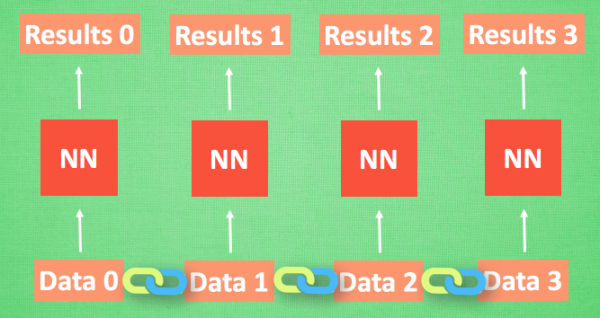那我们如何让数据间的关联也被 NN 加以分析呢? 想想我们人类是怎么分析各种事物的关联吧, 最基本的方式,就是记住之前发生的事情. 那我们让神经网络也具备这种记住之前发生的事的能力. 再分析 Data0 的时候, 我们把分析结果存入记忆. 然后当分析 data1的时候, NN会产生新的记忆, 但是新记忆和老记忆是没有联系的. 我们就简单的把老记忆调用过来, 一起分析. 如果继续分析更多的有序数据 , RNN就会把之前的记忆都累积起来, 一起分析.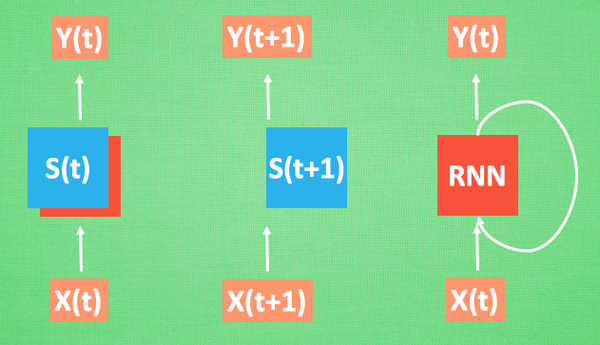我们再重复一遍刚才的流程, 不过这次是以加入一些数学方面的东西. 每次 RNN 运算完之后都会产生一个对于当前状态的描述 , state. 我们用简写 S(t) 代替, 然后这个 RNN开始分析 x(t+1) , 他会根据 x(t+1)产生s(t+1), 不过此时 y(t+1) 是由 s(t) 和 s(t+1) 共同创造的. 所以我们通常看到的 RNN 也可以表达成这种样子.

# 二、代码案例：

## 1.相关包

import torch
from torch import nn
import numpy as np
import matplotlib.pyplot as plt

## 2.网络结构

time_step和input_size的关系：每一个time_step，也就是每一次时间节点，都会读取input_size大小的数据。例如本次案例中，steps = np.linspace(start, end, 10, dtype=np.float32)，每一步随机给出10个数据点，而input_size=1，也就是每一次时间节点读取1个数据，而共有10个数据点，因此time_step=10.

# Hyper Parameters
TIME_STEP = 10      # rnn time step
INPUT_SIZE = 1      # rnn input size
LR = 0.02           # learning rate

class RNN(nn.Module):
def __init__(self):
super(RNN,self).__init__()
self.rnn=nn.RNN(
input_size=1, #输入特征值
hidden_size=32,#隐藏层
num_layers=1,#有几层RNN layers
batch_first=True## input & output 会是以 batch size 为第一维度的特征集 e.g. (batch, time_step, input_size)
)
self.out=nn.Linear(32,1)
def forward(self,x,h_state):
# x (batch, time_step, input_size)
# h_state (n_layers, batch, hidden_size)
# r_out (batch, time_step, output_size)
r_out,h_state=self.rnn(x,h_state)  #会输出目标值，还有状态值
outs=[]#保存所有时间点的预测值
for time_step in range(TIME_STEP):
outs.append(self.out(r_out[:,time_step,:]))
# r_out, h_state = self.rnn(x, h_state)
# r_out = r_out.view(-1, 32)
# outs = self.out(r_out)
# return outs.view(-1, 32, TIME_STEP), h_state

## 3.训练及其可视化

rnn=RNN()
optimizer = torch.optim.Adam(rnn.parameters(), lr=LR)   # optimize all rnn parameters
loss_func = nn.MSELoss()

h_state = None   # 要使用初始 hidden state, 可以设成 None

plt.ion()
for step in range(100):
start, end = step * np.pi, (step+1)*np.pi   # time steps
# sin 预测 cos
steps = np.linspace(start, end, 10, dtype=np.float32)
x_np = np.sin(steps)    # float32 for converting torch FloatTensor
y_np = np.cos(steps)

x = torch.from_numpy(x_np[np.newaxis, :, np.newaxis])    # shape (batch, time_step, input_size)
y = torch.from_numpy(y_np[np.newaxis, :, np.newaxis])

prediction, h_state = rnn(x, h_state)   # rnn 对于每个 step 的 prediction, 还有最后一个 step 的 h_state
# !!  下一步十分重要 !!
h_state = h_state.data  # 要把 h_state 重新包装一下才能放入下一个 iteration, 不然会报错

loss = loss_func(prediction, y)     # cross entropy loss
plt.plot(steps,prediction.data.numpy().flatten(),'b-')#因为最后prediction的shape为（1,10,1），需要压缩
plt.plot(steps,y_np,'r-')
plt.draw()
plt.pause(0.1)
plt.ioff()
plt.show()

可视化输出结果：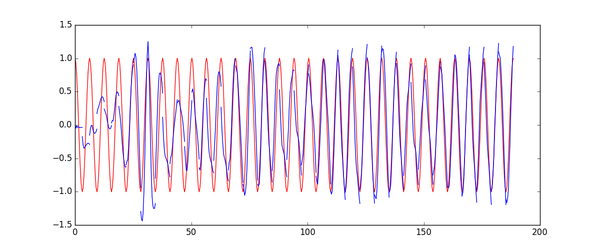展开全文深度学习 pytorch
• pytorch学习-7：RNN 循环神经网络（分类）1. 加载MNIST手写数据1.1 数据预处理2. RNN模型建立3. 训练4. 预测参考 循环神经网络让神经网络有了记忆, 对于序列话的数据,循环神经网络能达到更好的效果. 1. 加载MNIST...pytorch MNIST
• 因此，就有了现在的循环神经网络，他的本质是：拥有记忆的能力，并且会根据这些记忆的内容来进行推断。因此，他的输出就依赖于当前的输入和记忆。 网络结构及原理 循环神经网络的基本结构特别简单，就是将网络的输出...
• 原文中有更详尽的对RNN原理的介绍，形象生动，值得一看。 这个RNN模型的目的是计算两个八位二进制数的和，有进位。神经网络 numpy
• 最近又忍不住把RNN这一块儿的东西...关于RNN循环神经网络的具体概念我就不细讲了，稍微把概念给提下吧，然后会说说其变形模型，以及会给出两个demo讲述其在不同领域的应用，方便大家理解！ 1. RNN循环神经网络介绍 ...python
• 关键词：非图像识别，RNN，TensorFlow，代码，句子分类，NLP、 【注意】这是一个测试数据没有实际意义，所以需要实际数据的请看我的其他博客里有说明 有问题的留言问我就好。 最近在看RNN的句子分类，发现网上...tensorflow 情感分析 词向量
• rnn的matlab代码实现 ...一个使用Java实现的简单LSTM神经网络，在其中有多处优化，代码重构自另一个人的项目，但是链接我给丢了（WTF） 训练速度还可以，精度一般。 支持Matlab接口，记得加入classpath。matlab
• 目录 前言 一、普通神经网络 二、循环神经网络 1.前向传播 ...前段时间实验室人手一本《Deep Learning》，本文章结合这本圣经和博客上各类知识以及我自己的理解和实践，针对RNN循环神经网络作出...
• ## 循环神经网络RNN

千次阅读 2022-02-17 14:26:51
RNN（Recurrent Neural Network）循环神经网络。 类比血液在体内循环，从过去一直被更新到现在。 RNN具有环路。这个环路可以使数据不断循环。通过数据的循环，RNN一边记住过去的数据，一边更新到最新的数据。 RNN层...
• RNN循环神经网络与时空序列数据 RNN全称循环神经网络（Recurrent Neural Networks），是用来处理时空序列数据的。语言、文章、股票走势这些都是常见的序列信号，我们交谈的时候要听懂一句话的内容，首先要依次听懂...自然语言处理 机器学习 深度学习 tensorflow
• 深度学习框架TensorFlow视频培训课程概况：涉及TensorFlow的安装、基本语法、TensorFlow的使用、训练、回归模型、使用TensorFlow构建神经网络，线性回归、Mnist数据集、逻辑回归、卷积神经网络、循环神经网络、项目...tensorflow 深度学习 人工智能
•matlab
• 基于循环神经网络RNN）预测分子性质 导入库 from rdkit import Chem from rdkit.Chem.Crippen import MolLogP import numpy as np import torch import time 载入数据，计算分子指纹和描述符 maxlen = 64 with ...
• NeuralNetStudio：开源递归神经网络程序（RNN）。 [MATLAB]
• 循环神经网络是一种时间序列预测模型，多应用在自然语言处理上。 原理网上是有很多的，不展开解释，本文基于一个二进制加法，进行python实现。 其实python代码并非本人实现 具体参考两篇博客，第一篇是英语原文，...深度学习
• 深度学习的顶级循环神经网络的工作方式包括 LSTM、GRU 和 RNN. 循环神经网络(RNN)在自然语言处理、语音识别等有很广泛的用途。LSTM和GRU是目前使用最广泛的两个循环神经网络的模型变种。该视频课程内容主要分为三大...nlp LSTM GRU NLP
• 主要为大家详细介绍了基于循环神经网络(RNN)实现影评情感分类，具有一定的参考价值，感兴趣的小伙伴们可以参考一下
• 基于基本的RNN的Python代码，将其用Matlab实现了，且实验结果比较好...